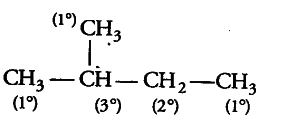# The relative reactivity of 1°, 2°, 3° hydrogens towards chlorination is 1: 3.8: 5

The relative reactivity of 1°, 2°, 3° hydrogens towards chlorination is 1: 3.8: 5. Calculate the percentages of all monochlorinated products obtained from 2-methylbutane.Relative amount of monochlorinated product = Number of hydrogen X relative reactivity For (1°) monochlorinated product = 9x1 = 9
(2°) monochlorinated product = 2x 3.8 = 7.6 (3°) monochlorinated
product = 1x3 = 5
Total amount of monochlorinated compounds = 9 + 7.6+ 5 = 21.6

% of 1° monochlorinated product = 9x100/21.6 =41.67
% of 2° monochlorinated product =7.6x100/21.6 =35.18
% of 3° monochlorinated product =5x100/21.6 =23.15